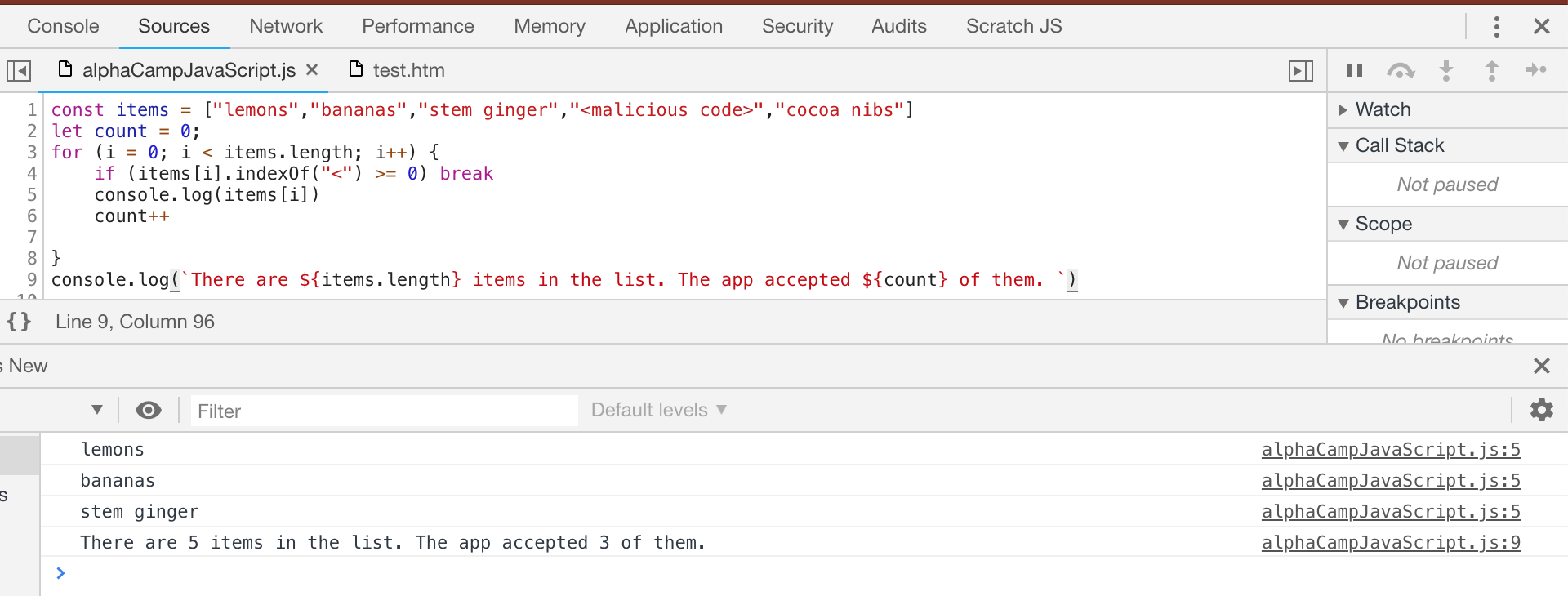# Loop 迴圈

• while：只要條件有效，就持續執行
• for：控制執行次數

## 只要條件有效，就持續執行：While 迴圈

``````let x = 1
while (x <= 3) {
console.log(`I have looped \${x} time(s)...`)
x++
}
console.log('Finished!')

``````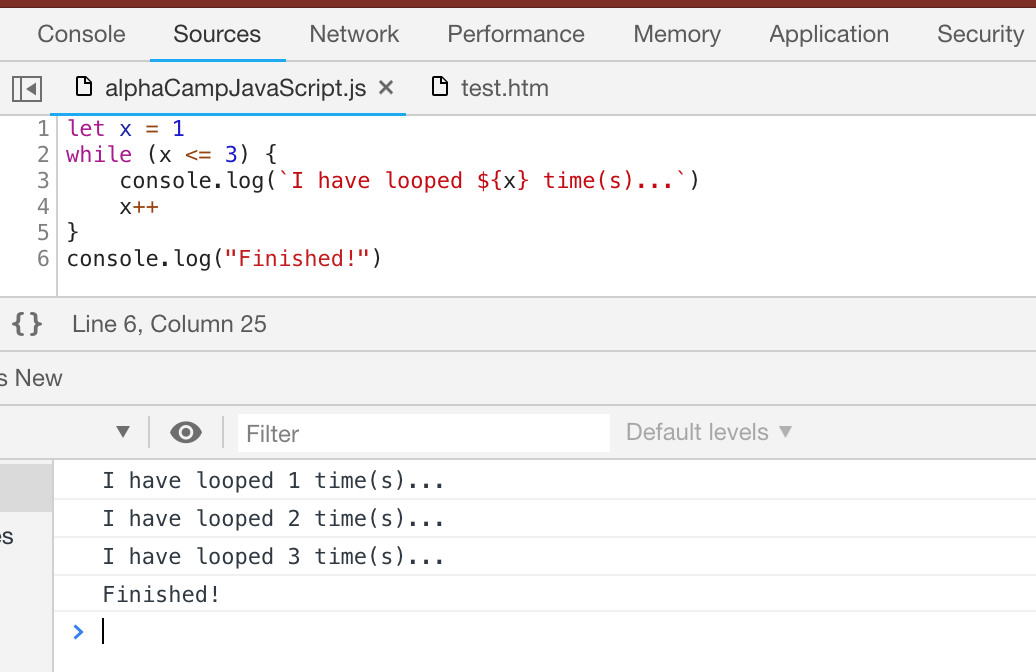• 關鍵字`while`
• 條件：在 `( )` 裡面定義條件，只要條件表達式 (expression) 回傳 true，就會執行 `{}` 裡的程序；
• 執行程序：將你希望重複的動作定義在 `{ }`之中。

## 無限迴圈 (infinite loop)

``````while (true) {
console.log('This loop is running.....')
}
``````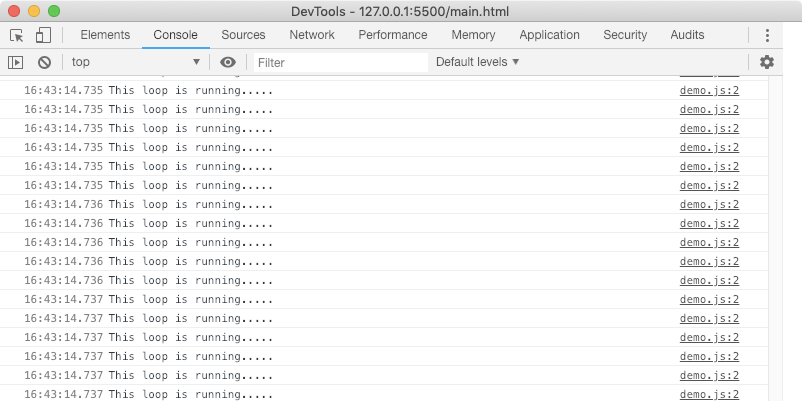## 控制執行次數：for 迴圈

``````for (let x = 1; x <= 3; x++ ) {
console.log(`I have looped \${x} time(s)...`)
}
console.log('Finished!')
``````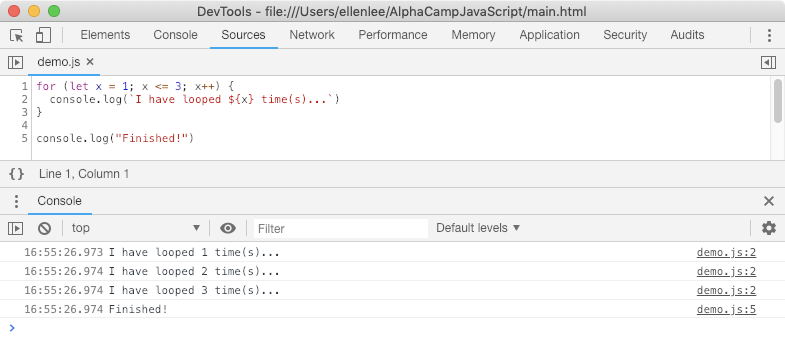• 關鍵字`for`
• 條件：在 `( )` 裡面需要定義三種資訊：
1. 宣告索引變數，請注意需要關鍵字如 `let x = 1`

2. 停止條件，如 `x <= 3`，在此條件為 true 之前，迴圈都會執行；

3. 每次迭代的變化，如 `x++` 讓迴圈每執行一次，做為索引的變數 `x` 就會加 1。

• 執行程序：將你希望重複的動作定義在 `{ }`之中。

## For 迴圈與陣列

``````const weekDays = ['Mánudagur', 'Þriðjudagur', 'Miðvikudagur', 'Fimmtudagur', 'Föstudagur', 'Laugardagur', 'Sunnudagur' ]
``````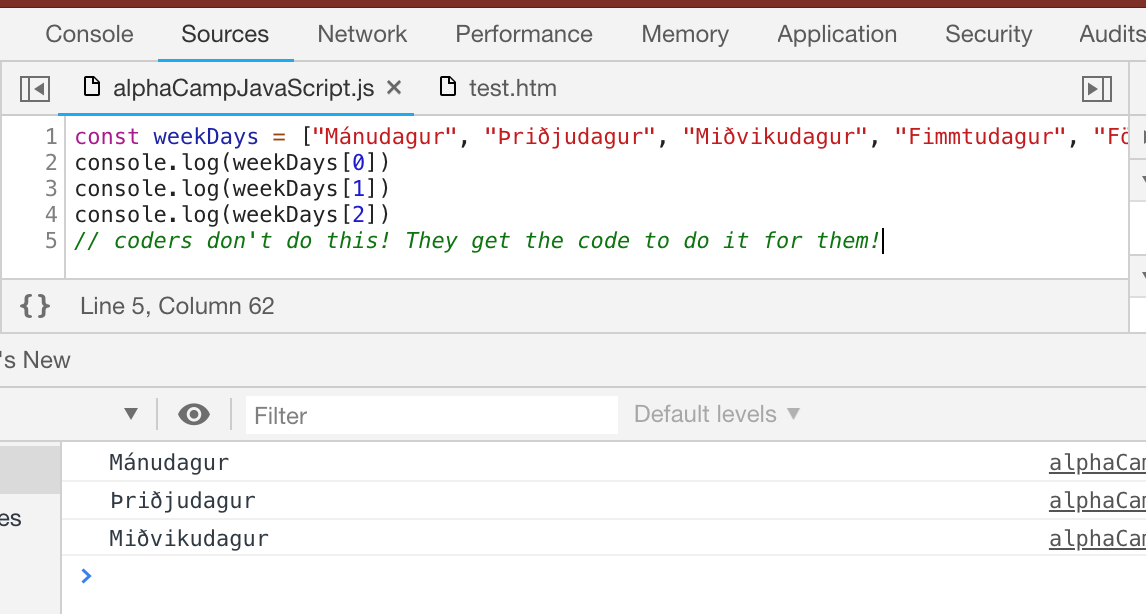``````for (let x = 0; x < weekDays.length; x++) {
console.log(weekDays[x])
}
``````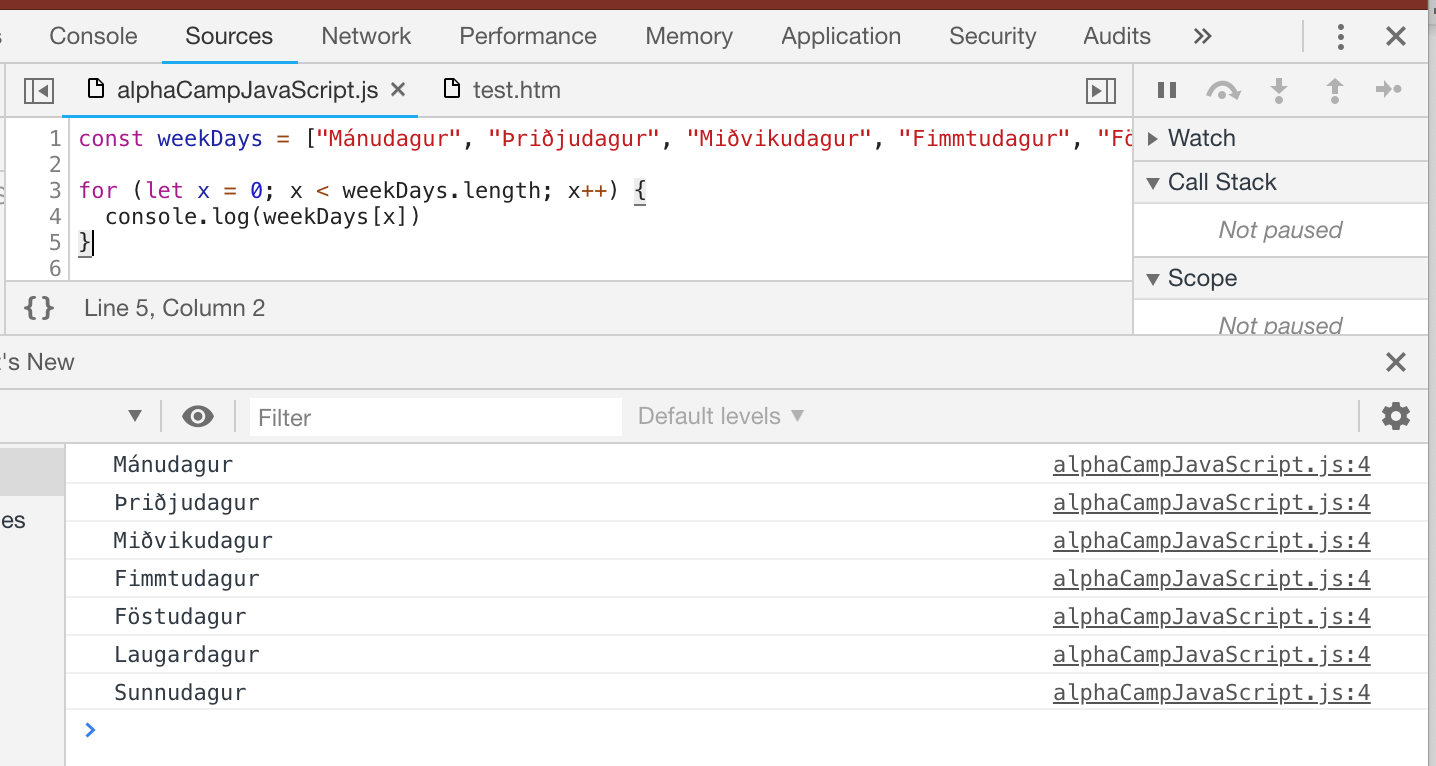``````for (let x = weekDays.length - 1; x >= 0 ; x--) {
console.log(`\${weekDays[x]} is day \${x + 1}`)
}
``````## 跳脫迴圈：break

``````let items = ['lemons','bananas','stem ginger','<malicious code>','cocoa nibs']

let items = ['lemons','bananas','stem ginger','<malicious code>','cocoa nibs']
for (i = 0; i < items.length; i++) {
console.log(items[i])
}
``````

• 用 `"<"` 當成檢查的目標，如果 `"<"` 存在，就表示遇到惡意的內容，我們會使用`_string_.indexOf(_string_)` 方法，它會在一個字串中搜尋另一個字串，如果它沒找到相對應的字串，會回傳 -1；
• 跳出迴圈，使用 break

``````const items = ['lemons','bananas','stem ginger','<malicious code>','cocoa nibs']
let count = 0;
for (i = 0; i < items.length; i++) {
if (items[i].indexOf('<') >= 0) break
console.log(items[i])
count++
}
console.log(`There are \${items.length} items in the list. The app accepted \${count} of them. `)
``````